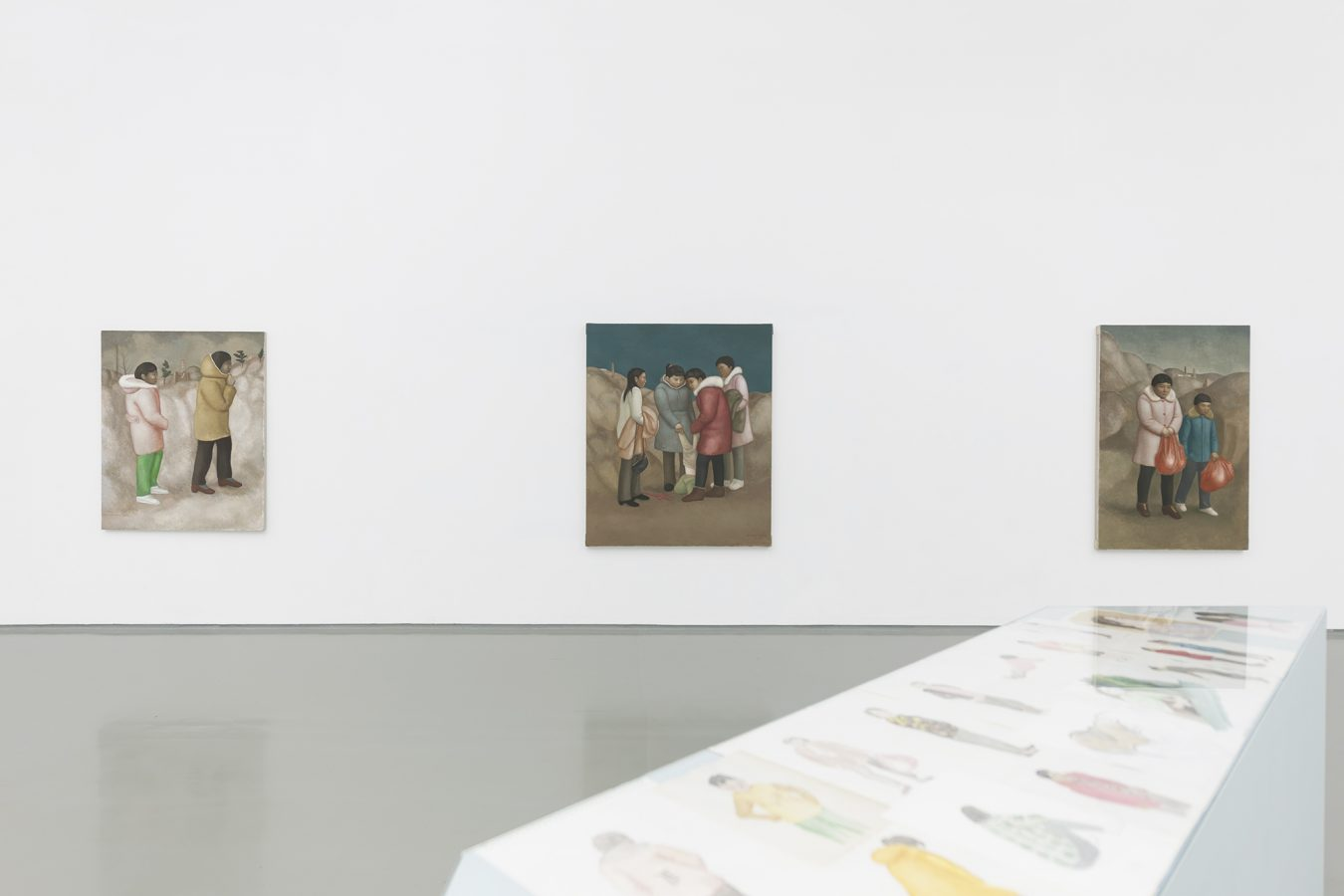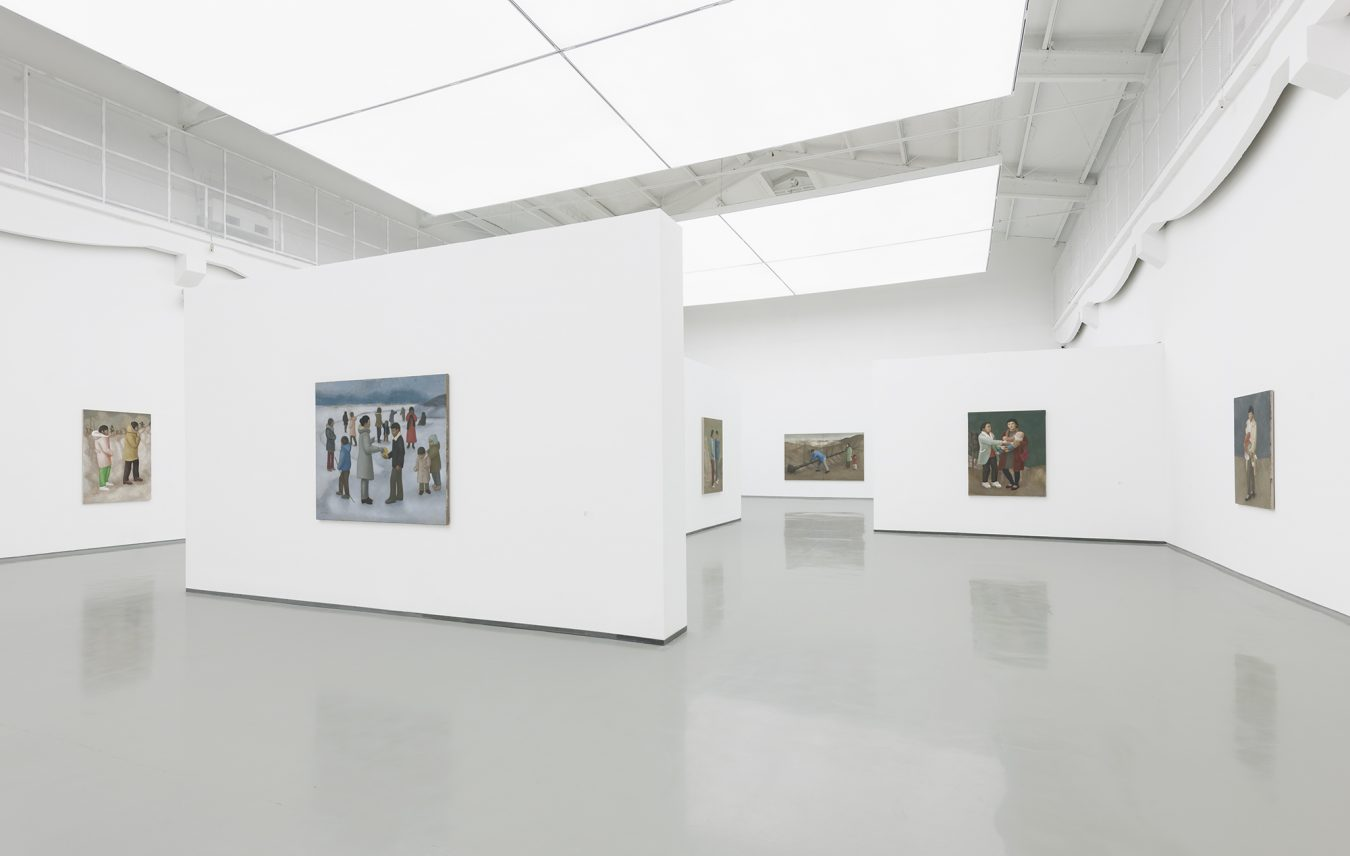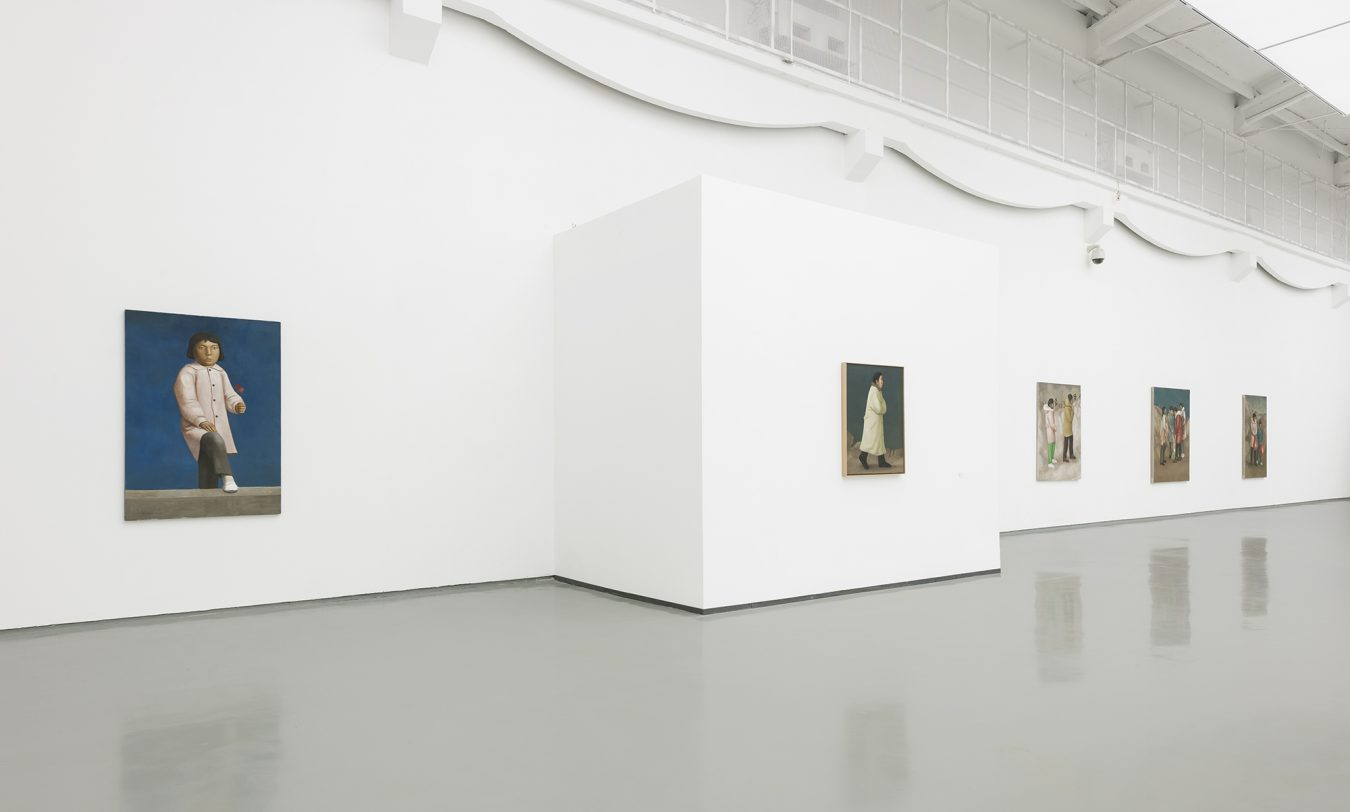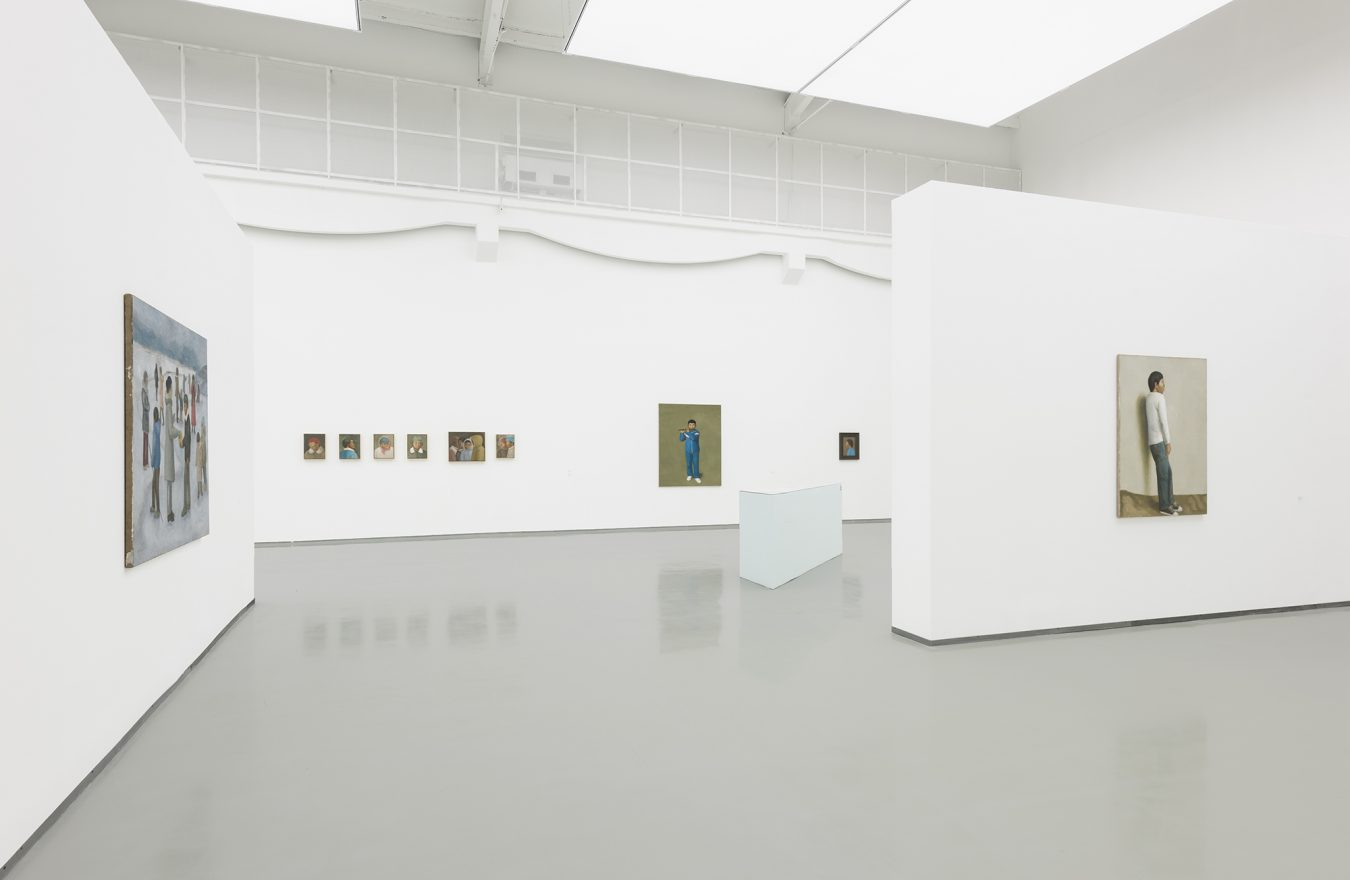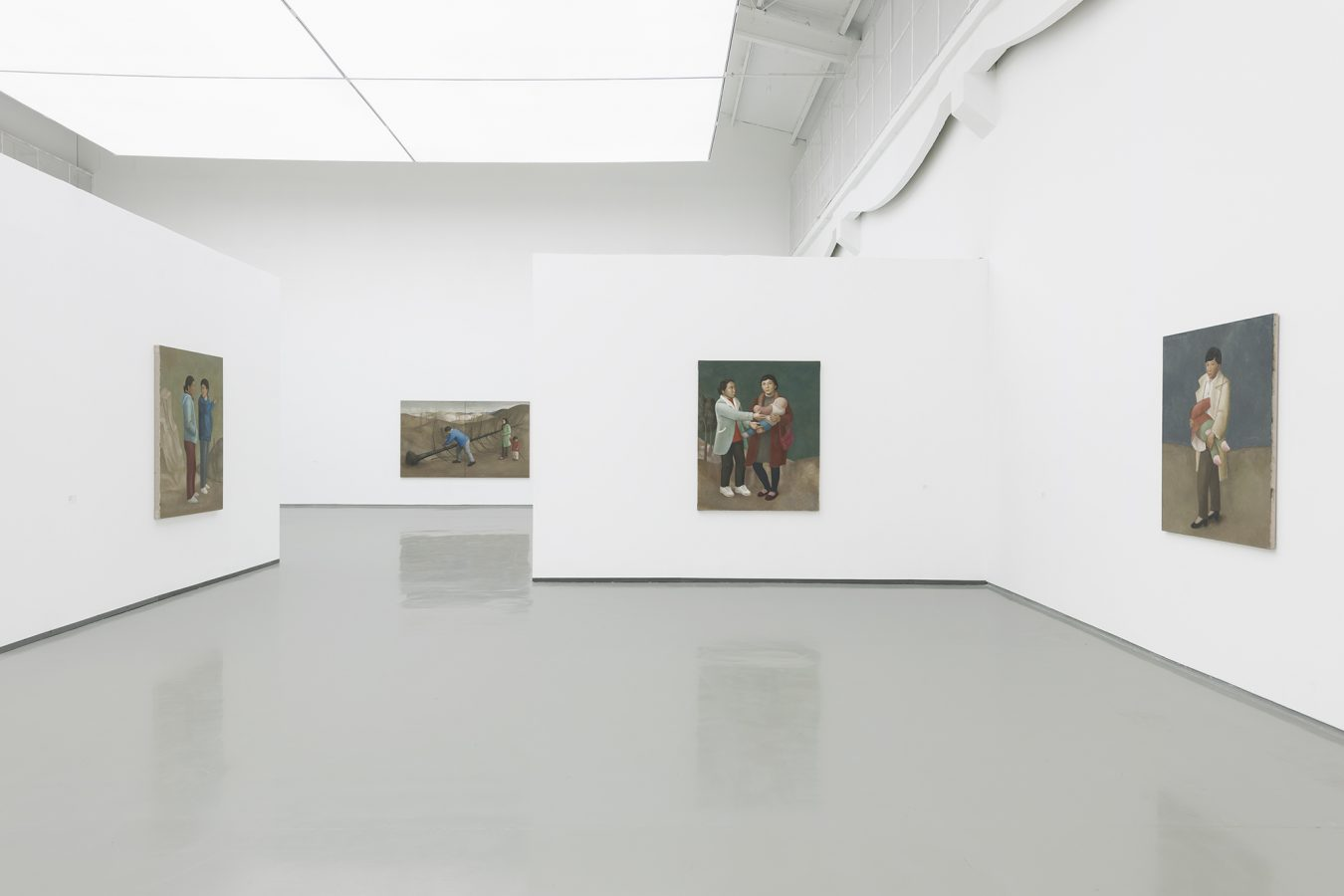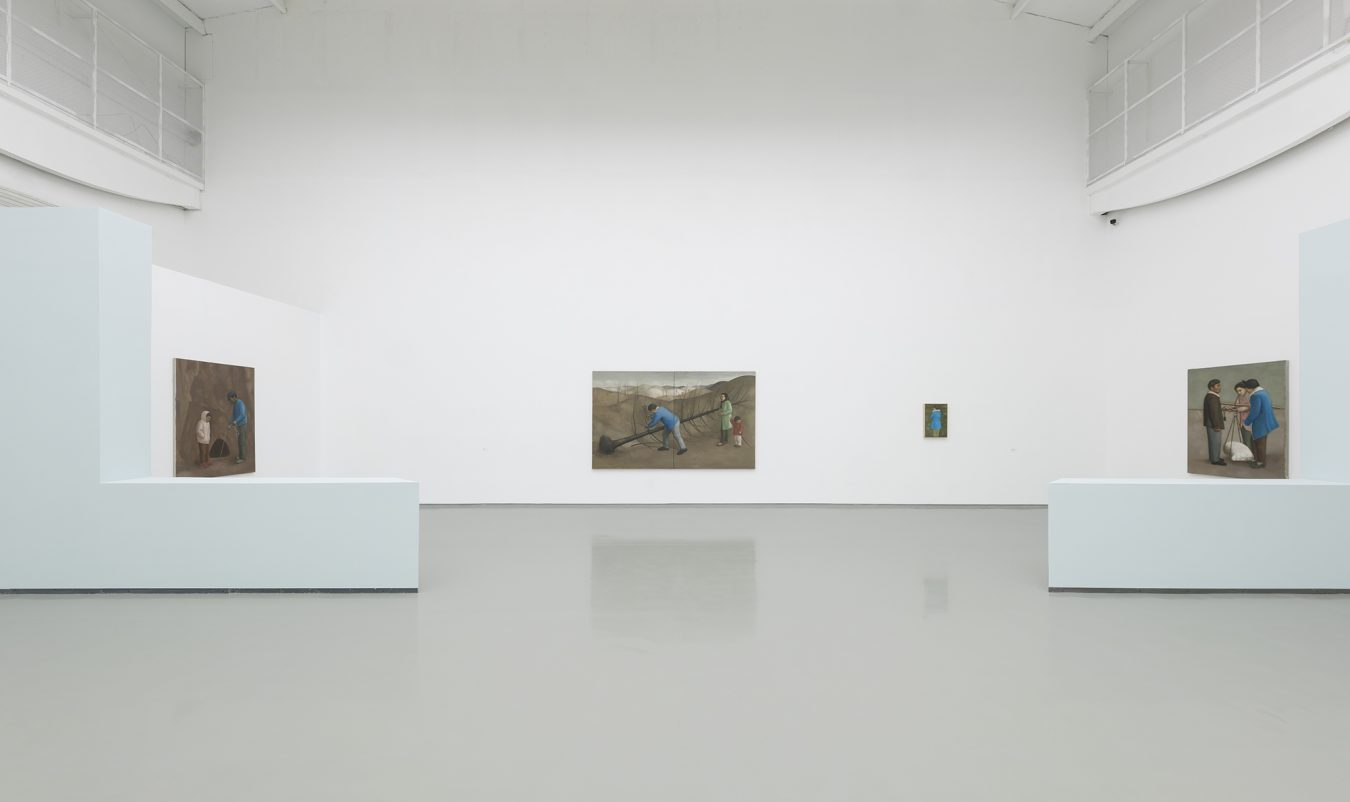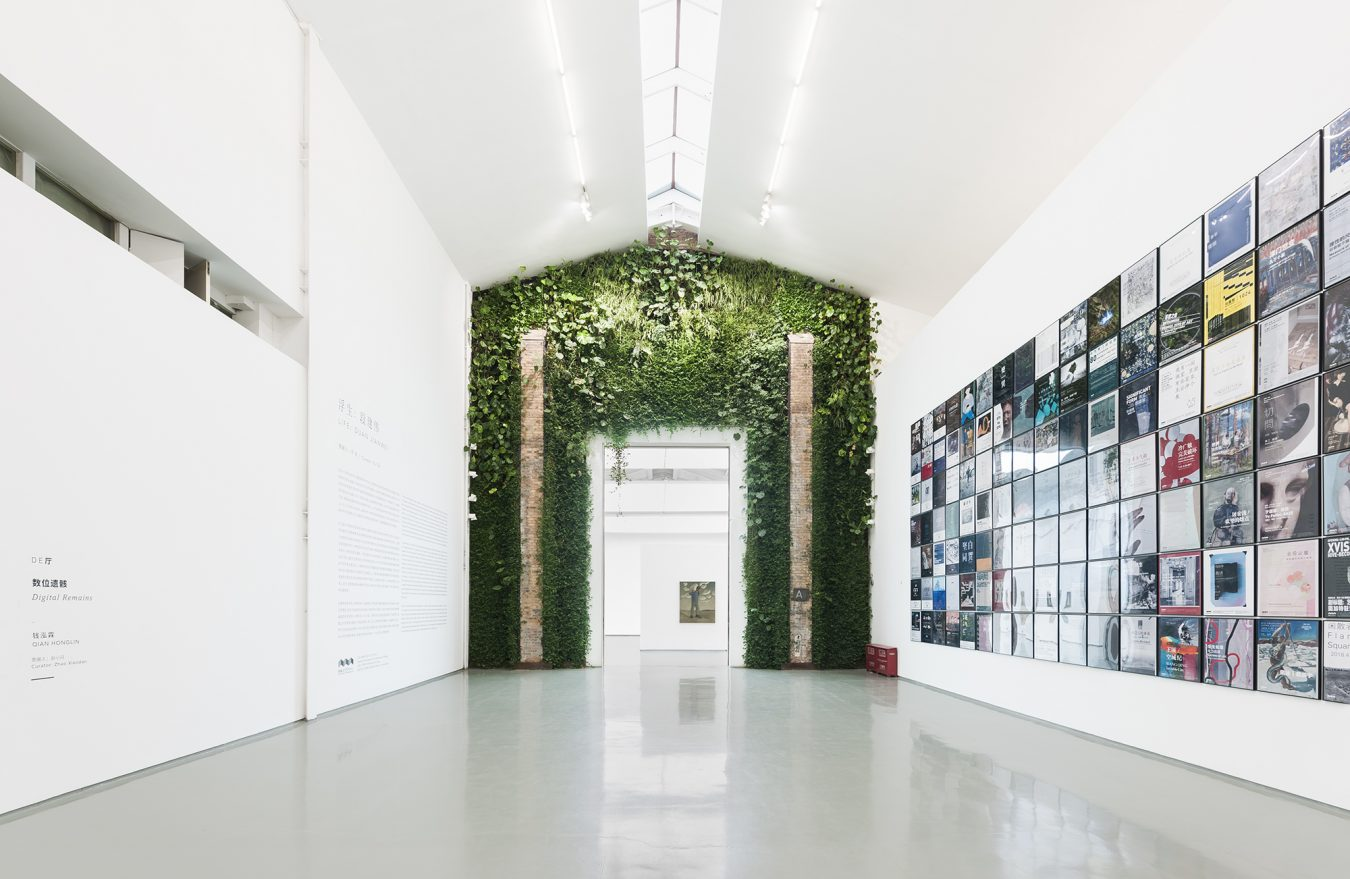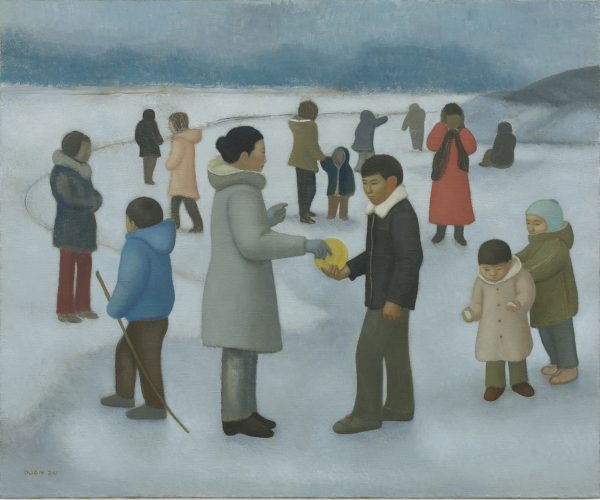2020. 布面油画. 140×170cm.2020. 布面油画. 40×30cm.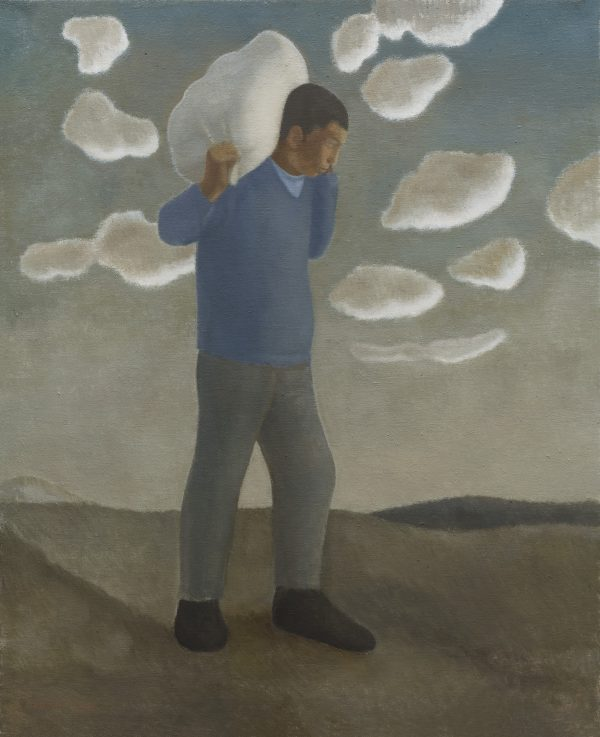2019. 布面油画. 135×110cm.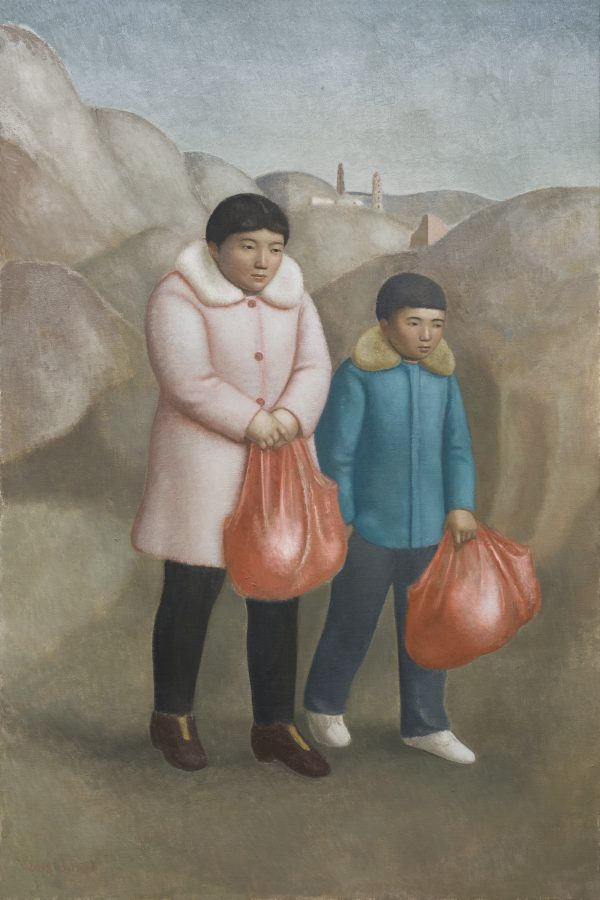2018. 布面油画. 180×120cm.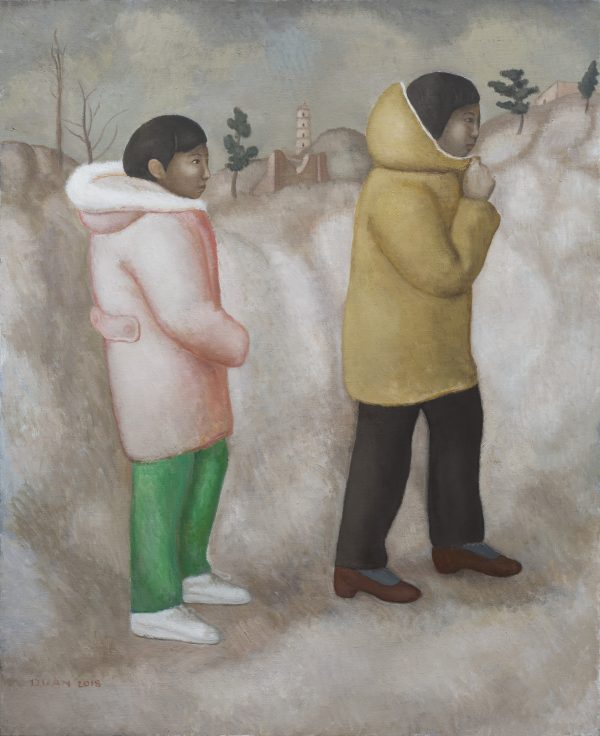2018. 布面油画. 160×130cm.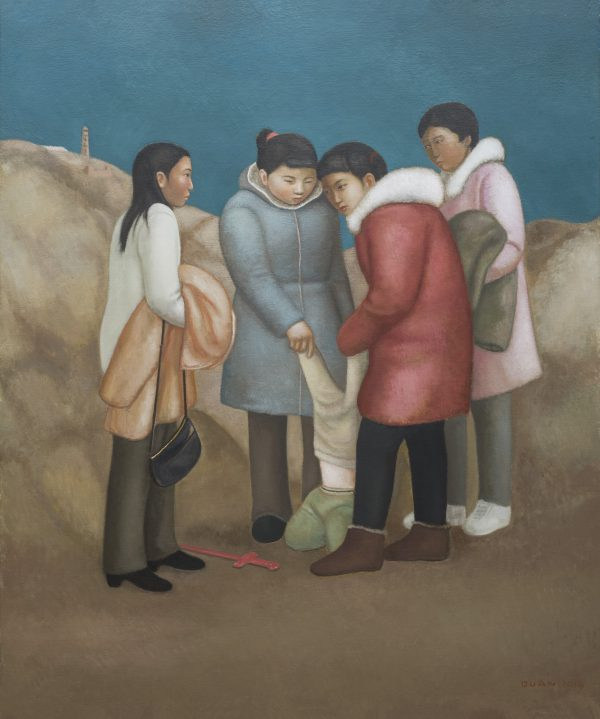2018. 布面油画. 180×150cm.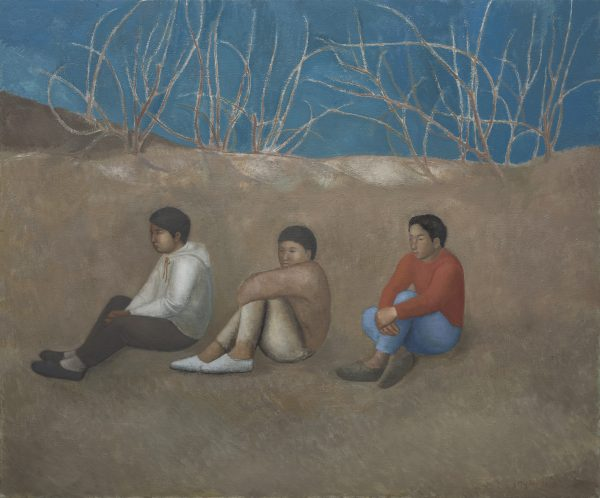2018. 布面油画. 150×180cm.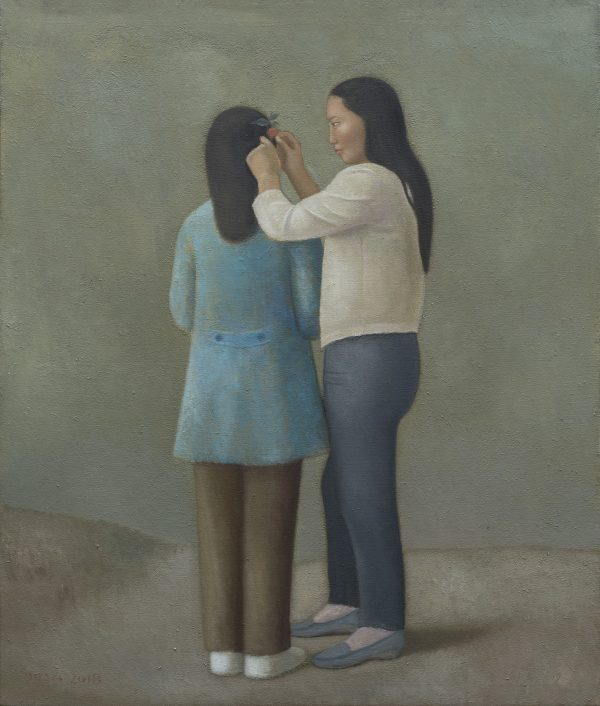2018. 布面油画. 130×110cm.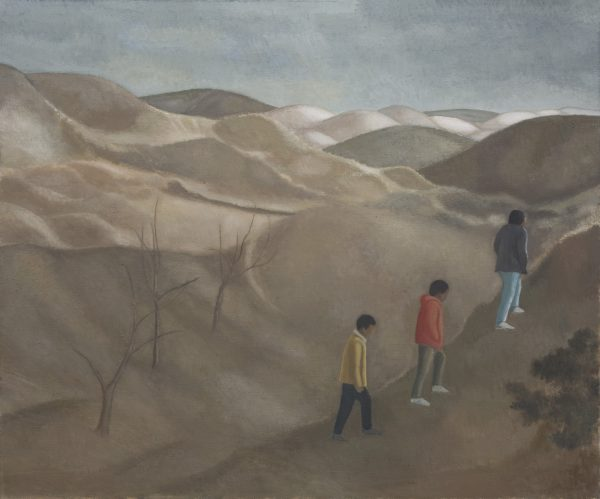2018. 布面油画. 150×180cm.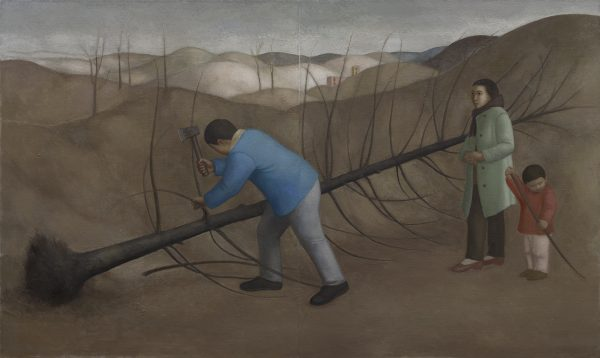2018. 布面油画. 180×300cm.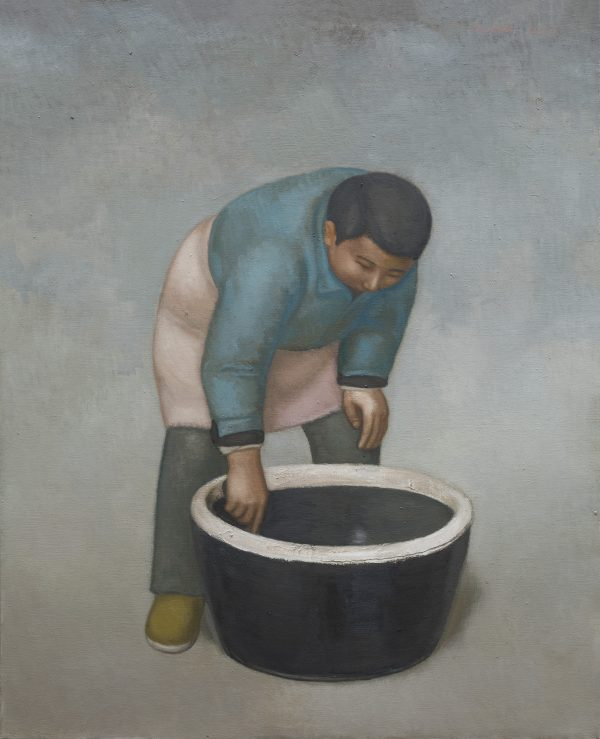2017. 布面油画. 135×110cm.2017. 布面油画. 135×110cm.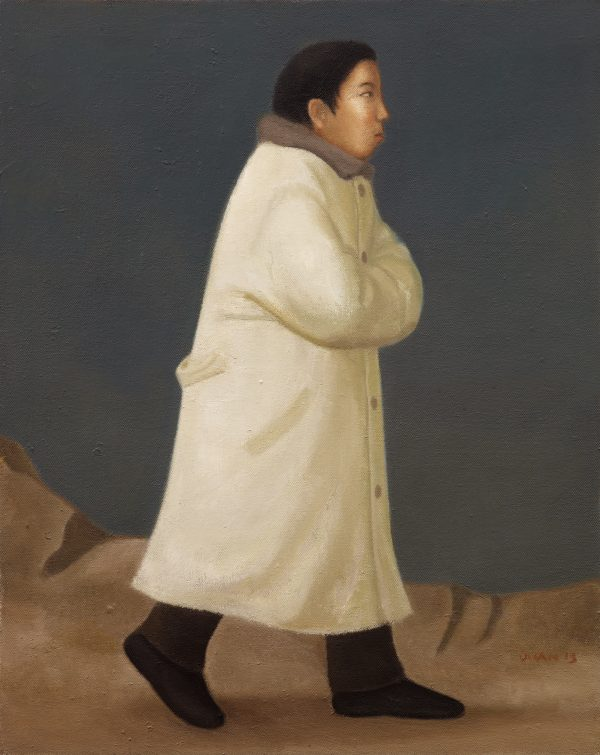2013. 布面油画. 100×80cm.2012. 布面油画. 160x110cm.